# 1757 A Shillelagh Puzzle

### Today’s Puzzle:

When I was in elementary school, one of my teachers had us listen to a variety of songs for Saint Patrick’s Day including Bing Crosby’s It’s the Same Old Shillelagh. It’s almost Saint Patrick’s Day, so here’s a puzzle that looks a little like a shillelagh, an Irish walking stick.

Since this is a level-3 puzzle, begin with the clues at the top of the puzzle and work your way down using each number 1 to 12 once in the first column and once in the top row. The numbers you write and the given clues need to form a multiplication table. There is only one solution.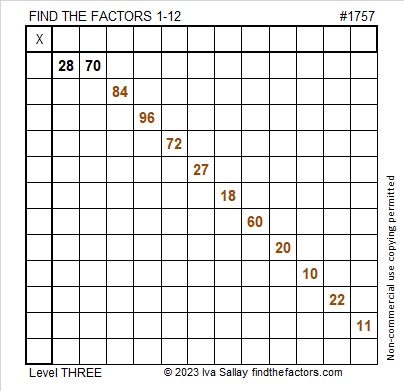Here is the same puzzle in black and white:### Factors of 1757:

• 1757 is a composite number.
• Prime factorization: 1757 = 7 × 251.
• 1757 has no exponents greater than 1 in its prime factorization, so √1757 cannot be simplified.
• The exponents in the prime factorization are 1 and 1. Adding one to each exponent and multiplying we get (1 + 1)(1 + 1) = 2 × 2 = 4. Therefore 1757 has exactly 4 factors.
• The factors of 1757 are outlined with their factor pair partners in the graphic below.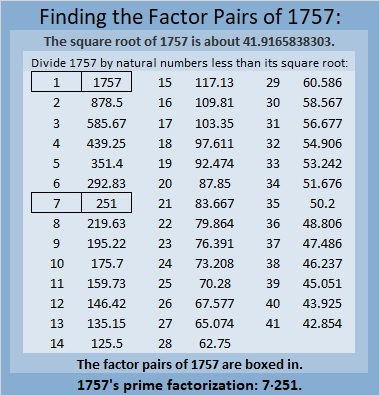### More About the Number 1757:

1757 is the difference of two squares in two different ways:
879² – 878² = 1757, and
129² – 122² = 1757.

1757 is palindrome 616 in base17
because 6(17²) + 1(17) + 6(1) = 1757.

# 1742 Just How Sharp Do You Think Cupid’s Arrow Is?

### Today’s Puzzle:

This puzzle looks a little like an arrow that Cupid might be aiming your way. I’m not sure how sharp it is, but here’s hoping that it’s not the least bit painful.

Since this is a level-3 puzzle, start with the clues at the top of the puzzle and work your way down cell by cell until you have found all the factors. Each number from 1 to 10 can only appear one time in the first column and one time in the top row. There is only one solution.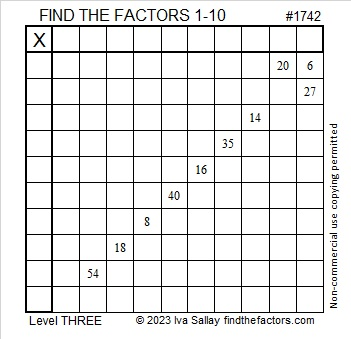### Factors of 1742:

• 1742 is a composite number.
• Prime factorization: 1742 = 2 × 13 × 67.
• 1742 has no exponents greater than 1 in its prime factorization, so √1742 cannot be simplified.
• The exponents in the prime factorization are 1, 1, and 1. Adding one to each exponent and multiplying we get (1 + 1)(1 + 1)(1 + 1) = 2 × 2 × 2 = 8. Therefore 1742 has exactly 8 factors.
• The factors of 1742 are outlined with their factor pair partners in the graphic below.### More About the Number 1742:

1742 is the hypotenuse of a Pythagorean triple:
670-1608-1742 which is (5-12-13) times 134.

1742 looks interesting in some other bases:
It’s 23432 in base 5 because 2(5⁴) + 3(5³) + 4(5²) + 3(5) + 2(1) = 1742,
2345 in base 9 because 2(9³) + 3(9²) + 4(9) + 5(1) = 1742, and
repdigit 222 in base 29 because 2(29² + 29 + 1) = 1742.

# 1732 Is a Thousand Times More Than…

### Today’s Puzzle:

If you know the common factor of 50 and 35 that will put only numbers from 1 to 12 in the first column and the top row of this multiplication table puzzle, then you will have completed the first step in solving the puzzle. Afterward, just work from the top of the puzzle to the bottom filling in factors as you go. That’s how you solve these level-three puzzles.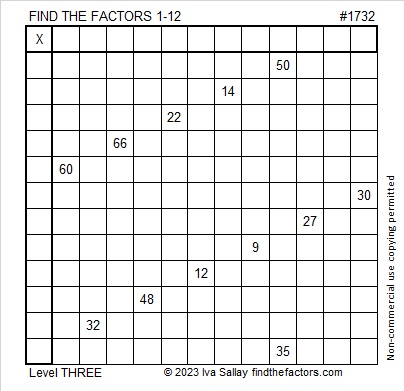### Factors of 1732:

• 1732 is a composite number.
• Prime factorization: 1732 = 2 × 2 × 433, which can be written 1732 = 2² × 433.
• 1732 has at least one exponent greater than 1 in its prime factorization so √1732 can be simplified. Taking the factor pair from the factor pair table below with the largest square number factor, we get √1732 = (√4)(√433) = 2√433.
• The exponents in the prime factorization are 2 and 1. Adding one to each exponent and multiplying we get (2 + 1)(1 + 1) = 3 × 2 = 6. Therefore 1732 has exactly 6 factors.
• The factors of 1732 are outlined with their factor pair partners in the graphic below.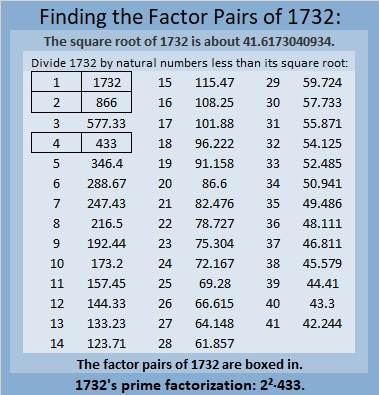### More About the Number 1732:

1732 is the sum of two squares:
34² + 24² = 1732.

1732 is the hypotenuse of a Pythagorean triple:
580-1632-1732, calculated from 34² – 24², 2(34)(24), 34² + 24².
It is also 4 times (145-408-433).

1732 is a palindrome in base 15 because
7(15²) + 10(15) + 7(1) = 1732.

I remember one of my college professors telling his class that
√2 is about 1.4, and Valentine’s day is February 14,
√3 is about 1.7, and Saint Patrick’s day is March 17.

That’s how I remember those values, but this tweet reminded me that 1732 is a thousand times more than the square root of three rounded to three decimal places. It also makes a reference to the square root of two.

# 1723 A Little Virgács

### Today’s Puzzle:

I haven’t blogged much this year so I guess I deserved a little bit of virgács in my shoes this morning. Mikulás (St. Nick) leaves virgács in the boots of naughty little boys or girls in the wee hours of December 6. Treats are for the good kids. What is virgács? It is small golden spray-painted twigs bound with some pretty red ribbon.  Of course, all children are sometimes naughty and sometimes nice, so they could all expect to get virgács along with their treats in their boots this morning.

You can solve this virgács puzzle by starting with the clues at the top of the grid, finding their factors, and working down the puzzle row by row until you have found all the factors. Each number from 1 to 10 must appear exactly one time in both the first column and the top row.### Factors of 1723:

• 1723 is a prime number.
• Prime factorization: 1723 is prime.
• 1723 has no exponents greater than 1 in its prime factorization, so √1723 cannot be simplified.
• The exponent in the prime factorization is 1. Adding one to that exponent we get (1 + 1) = 2. Therefore 1723 has exactly 2 factors.
• The factors of 1723 are outlined with their factor pair partners in the graphic below.How do we know that 1723 is a prime number? If 1723 were not a prime number, then it would be divisible by at least one prime number less than or equal to √1723. Since 1723 cannot be divided evenly by 2, 3, 5, 7, 11, 13, 17, 19, 23, 29, 31, 37, or 41, we know that 1723 is a prime number.

### More About the Number 1723:

1723 and 1721 are twin primes.

1723 is the sum of consecutive numbers:
861 + 862 = 1723.

1723 is the difference of consecutive squares:
862² – 861² = 1723.

# 1698 A Little Virgács and Candy

### Today’s Puzzle:

If you were a child in Hungary, you might have found some virgács and some candy in your boot this morning. Mikulás (St. Nick) would have given you the candy because of how good you’ve been this year, and the virgács for those times you weren’t so good.

This virgács and candy puzzle is like a mixed-up multiplication table. It is a lot easier to solve because I made it a level 3 puzzle. First, find the common factor of 56 and 72 that will allow only numbers between 1 and 12 to go in the first column. Put the factors in the appropriate cells, then work your way down the puzzle, row by row until each number from 1 to 12 is in both the first column and the top row.### Factors of 1698:

• 1698 is a composite number.
• Prime factorization: 1698 = 2 × 3 × 283.
• 1698 has no exponents greater than 1 in its prime factorization, so √1698 cannot be simplified.
• The exponents in the prime factorization are 1, 1, and 1. Adding one to each exponent and multiplying we get (1 + 1)(1 + 1)(1 + 1) = 2 × 2 × 2 = 8. Therefore 1698 has exactly 8 factors.
• The factors of 1698 are outlined with their factor pair partners in the graphic below.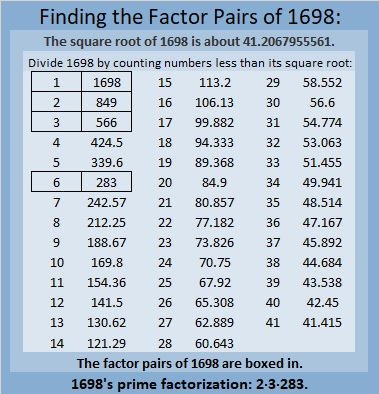### More About the Number 1698:

1698 = 2(849)(1), so it is a leg in the Pythagorean triple calculated from
2(849)(1), 849² – 1², 849² + 1².

1698 = 2(283)(3), so it is a leg in the Pythagorean triple calculated from
2(283)(3), 283² – 3², 283² + 3².

# 1686 Some Candy Corn for You to Chew on

### Today’s Puzzle:

Candy corn probably isn’t your favorite Halloween treat, but this candy corn puzzle could give you something satisfying to chew on. Give it a try!

Find the common factor of 33 and 66, write the factors in the appropriate cells. Since this is a level 3 puzzle, you can then work from the top of the puzzle row by row until you have found all the factors. The numbers from 1 to 12 must appear once in both the first column and the top row.Here’s the same puzzle without any color if you prefer: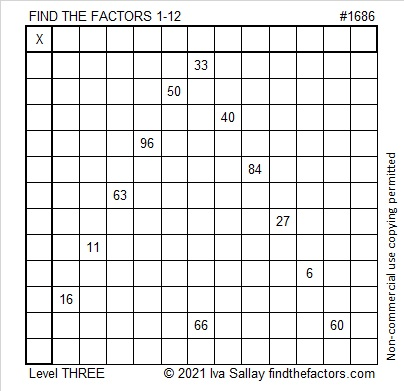### Factors of 1686:

• 1686 is a composite number.
• Prime factorization: 1686 = 2 × 3 × 281.
• 1686 has no exponents greater than 1 in its prime factorization, so √1686 cannot be simplified.
• The exponents in the prime factorization are 1, 1, and 1. Adding one to each exponent and multiplying we get (1 + 1)(1 + 1)(1 + 1) = 2 × 2 × 2 = 8. Therefore 1686 has exactly 8 factors.
• The factors of 1686 are outlined with their factor pair partners in the graphic below.### More about the number 1686:

1686 is the hypotenuse of a Pythagorean triple:
960-1386-1686, which is 6 times (160-231-281).

1686 is also a leg in these two Pythagorean triples:
1686-710648-710650, calculated from 2(843)(1), 843² – 1², 843² + 1² and
1686-78952-78970, calculated from 2(281)(3), 281² – 3², 281² – 3².

# 1675 You CAN Solve This Level 3 Puzzle!

Great news for math enthusiasts, students, and teachers everywhere! The #148 Playful Math Education Carnival was published today at Math Book Magic!

### Today’s puzzle:

I am confident that you can solve this level 3 puzzle! Here’s how: Using only the numbers from 1 to 10, write the factors of 27 and 6 in the appropriate cells. Next, write 18’s factors. Then, since this is a level 3 puzzle, write the factors of 30, 50, 40, 36, 14, 56, and 8, in that order, until all the numbers from 1 to 10 appear in the first column as well as in the top row. As always, there is only one solution.What did I tell you? You could solve it!

### Factors of 1675:

• 1675 is a composite number.
• Prime factorization: 1675 = 5 × 5 × 67, which can be written 1675 = 5² × 67.
• 1675 has at least one exponent greater than 1 in its prime factorization so √1675 can be simplified. Taking the factor pair from the factor pair table below with the largest square number factor, we get √1675 = (√25)(√67) = 5√67.
• The exponents in the prime factorization are 2 and 1. Adding one to each exponent and multiplying we get (2 + 1)(1 + 1) = 3 × 2 = 6. Therefore 1675 has exactly 6 factors.
• The factors of 1675 are outlined with their factor pair partners in the graphic below.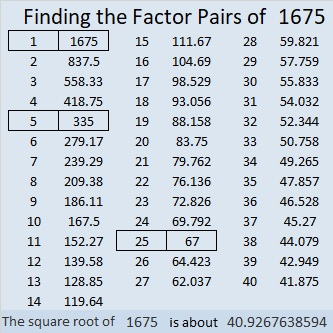### More About the Number 1675:

Did you notice that 1675 = 5 × 67 × 5?

How many quarters are in \$16.75?
Well, 17 × 4 quarters = 68 quarters = \$17.00.
Subtracting one quarter from both sides of the equation, we get
67 quarters = \$16.75.

1675 is the hypotenuse of TWO Pythagorean triples:
469-1608-1675, which is (7-24-25) times 67, and
1005-1340-1675, which is (3-4-5) times 335.

1675 is the difference of two squares in THREE different ways:
838² – 837² =  1675,
170² – 165² =  1675, and
46² – 21²  =  1675.

# 1666 Demystifying a Tricky Puzzle

### Today’s Puzzle:

This puzzle isn’t as tricky as it could be simply because I arranged its clues into a level 3 puzzle. That means that after you write the factors of 99 and 18 in the appropriate boxes in the first column and top row, you work your way down the puzzle clue by clue in the order they appear. Still, you will have to think about what to do with the 70, but I think you can handle it!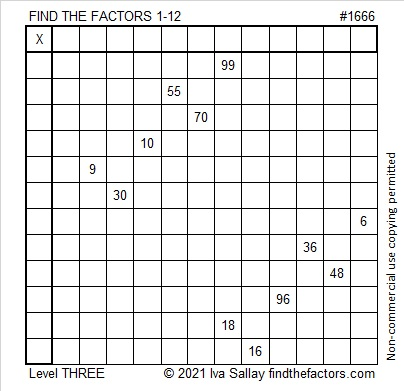### Factors of 1666:

• 1666 is a composite number.
• Prime factorization: 1666 = 2 × 7 × 7 × 17, which can be written 1666 = 2 × 7² × 17.
• 1666 has at least one exponent greater than 1 in its prime factorization so √1666 can be simplified. Taking the factor pair from the factor pair table below with the largest square number factor, we get √1666 = (√49)(√34) = 7√34.
• The exponents in the prime factorization are 1, 2, and 1. Adding one to each exponent and multiplying we get (1 + 1)(2 + 1)(1 + 1) = 2 × 3 × 2 = 12. Therefore 1666 has exactly 12 factors.
• The factors of 1666 are outlined with their factor pair partners in the graphic below.### More About the Number 1666:

1666 is the sum of two squares because ALL of its odd prime factors either leave a remainder of 1 when divided by 4 OR have an even exponent:
1666 = 2 × 7² × 17,
17÷4 = 4 R1, The exponent on 7² is even.
What are the two squares?
35² +  21² = 1666.

1666 is the hypotenuse of a Pythagorean triple :
784-1470-1666 which is (8-15-17) times 98 and
can also be calculated from 35² –  21², 2(35)(21), 35² +  21².

# 1652 Start at the Top and Work Your Way Down to the Bottom

### Today’s Puzzle:

This is a level 3 puzzle so the clues are given in a logical order starting from the top of the puzzle. Begin by writing the factors of 20 and 32 in the appropriate cells. Then write the rest of the numbers so that both the first column and the top row have all the numbers from 1 to 10, and the written numbers are the factors of the given clues.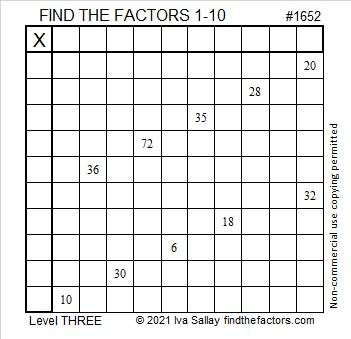### Factors of 1652:

• 1652 is a composite number.
• Prime factorization: 1652 = 2 × 2 × 7 × 59, which can be written 1652 = 2² × 7 × 59.
• 1652 has at least one exponent greater than 1 in its prime factorization so √1652 can be simplified. Taking the factor pair from the factor pair table below with the largest square number factor, we get √1652 = (√4)(√413) = 2√413.
• The exponents in the prime factorization are 2, 1, and 1. Adding one to each exponent and multiplying we get (2 + 1)(1 + 1)(1 + 1) = 3 × 2 × 2 = 12. Therefore 1652 has exactly 12 factors.
• The factors of 1652 are outlined with their factor pair partners in the graphic below.### More About the Number 1652:

1652 is the difference of two squares two different ways:
414² – 412² = 1652 and
66² – 52² = 1652.

# 1641 and Level 3

### Today’s Puzzle:

This is a level 3 puzzle so the clues have been placed so that you can know what order you should use the clues. Place the factors of 90 and 30 in the appropriate cells, then work your way down the puzzle cell by cell filling in the factors of the clues as you go.### Factors of 1641:

1 + 4 + 1 = 6, so 1641 is divisible by 3. (It isn’t necessary to include multiples of 3 in the sum to determine divisibility by 3.)

• 1641 is a composite number.
• Prime factorization: 1641 = 3 × 547.
• 1641 has no exponents greater than 1 in its prime factorization, so √1641 cannot be simplified.
• The exponents in the prime factorization are 1 and 1. Adding one to each exponent and multiplying we get (1 + 1)(1 + 1) = 2 × 2 = 4. Therefore 1641 has exactly 4 factors.
• The factors of 1641 are outlined with their factor pair partners in the graphic below.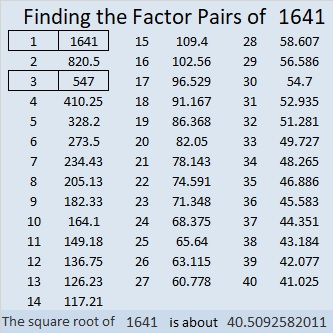### More About the Number 1641:

1641 is the difference of two squares in two different ways:
821² – 820² = 1641, and
275² – 272² = 1641.

From OEIS.org we learn that the number formed from 1²6²4²1² is a perfect square:
Recall that 1² = 1; 6² = 36; 4² = 16; and 1² = 1. Those squares form the number, 136161.
Sure enough, 136161 = 369².